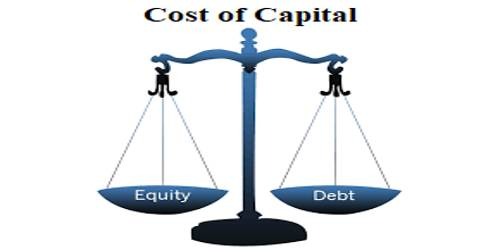# Importance and Necessity of Cost of Capital

Cost of capital: The cost of capital or required rate for return a firm can be defined as the composite cost of the firm’s financing components. The cost of capital is the rate of return a firm must earn on its investments in the project in order to maintain the market value of its.

The total capital at firm typically consists of several components and each component has its own cost. The idea of a cost of capital is to ‘bring these component costs together in one number the firm can use for the decision-making process. It is an important component of business valuation work.

There is two very important use of the cost of capital stand out particularly. These are given below –

(i) The cost of capital is an important link in achieving the financial goal of the firm -maximizing the stockholders’ wealth by maximizing the firm’s equity value.

(ii) The cost of capital is used to make capital budgeting decisions. It is important to use the correct minimum acceptable rate of return on new investments when making these decisions.

Importance of cost of capital: There are many importance costs of capital. It is the required return essential to make a capital budgeting project, such as building a new factory, worthwhile. Some are given below –

• Determination of capital structures;
• Project evaluation;
• Selecting sours of capital;
• Reducing cost;
• Determination of Discount rate;
• Declaration of dividend;
• Distribution of finding;
• Control of the firm;
• Evaluation of financial activities;
• Valuation of firms,
• Target profit.